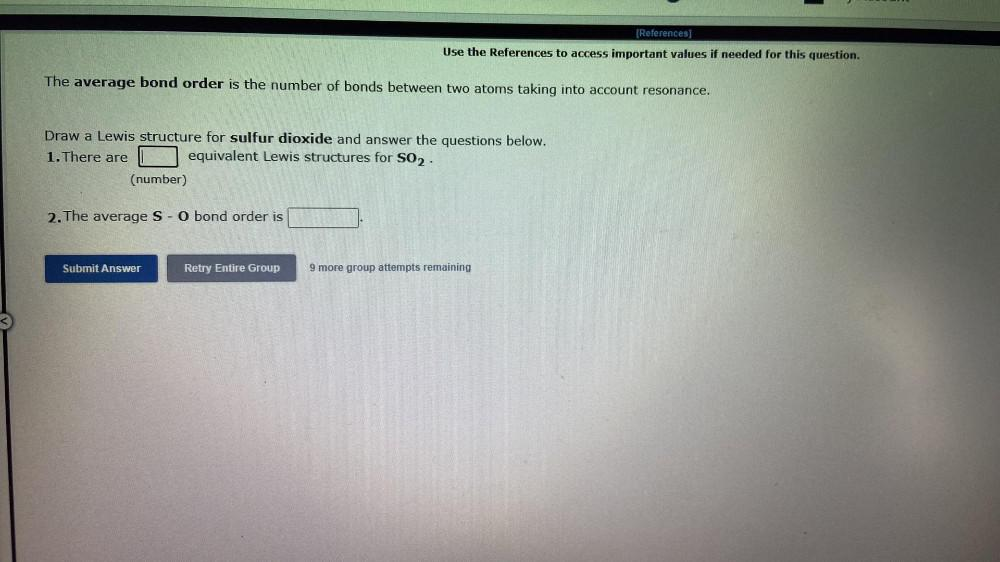Question:

# [References] Use the References to access important values if needed for this question. The average bond order is the number of[References] Use the References to access important values if needed for this question. The average bond order is the number of bonds between two atoms taking into account resonance. Draw a Lewis structure for sulfur dioxide and answer the questions below. 1. There are equivalent Lewis structures for S02. (number) 2. The average S - O bond order is Submit Answer Retry Entire Group 9 more group attempts remaining